# Math NodesMath nodes

The Math Nodes allow you to execute mathematical operations on values of type NUMBER, POINT and RGBO:

Number

The Number nodes allow you to execute operations on values of type NUMBER.

•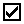Boolean
•Boolean Not
•Boolean And
•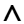Boolean Or
•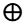Boolean Xor
•Number
•Number Add
•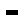Number Subtract
•Number Multiply
•Number Divide
•Number Power
•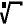Number Root
•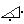Number XY Angle
•Number Sine
•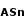Number ArcSine
•Number Cosine
•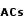Number ArcCosine
•Number Tangent
•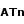Number ArcTangent
•Number Absolute
•Number Sign
•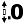Number Round
•Number Ceil
•Number Floor
•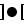Number Clamp

Point

The Point nodes allow you to execute operations on values of type POINT.

•Point
•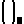Point Element
•Point Add
•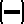Point Subtract
•Point Multiply
•Point Divide
•Point Scale
•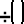Point Inverse Scale
•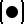Point Dot Product
•Point Cross Product
•Point Vector Normalize
•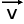Point Vector Length

Rgbo

The Rgbo nodes allow you to execute operations on values of type RGBO.

•Rgbo
•Rgbo Element
•Rgbo From Numbers
•Rgbo Add
•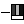Rgbo Subtract
•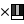Rgbo Multiply
•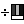Rgbo Divide
•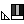Rgbo Scale
•Rgbo Apply Opacity
•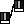Rgbo Lerp

Main Page